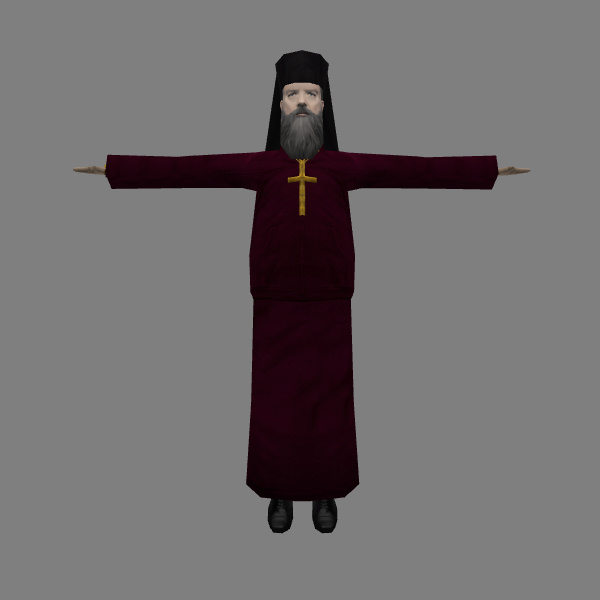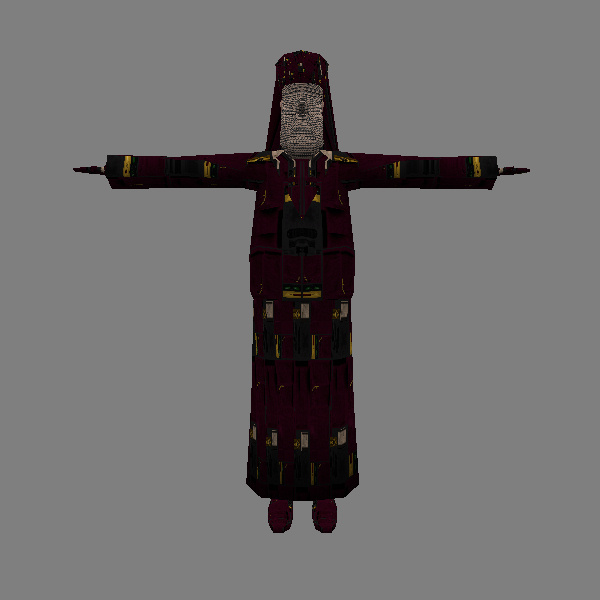# Converting Xash3D model UV coordinates to GoldSource coordinates Created 9 months ago2021-11-08 10:57:09 UTC byvenko

Created 9 months ago2021-11-08 10:57:09 UTC by

Posted 9 months ago2021-11-08 10:57:09 UTC Post #346042
Hi.
So, Xash3D uses a new "half-float" UV texture coordinates inside the smd/mdl, while goldsource uses the old "fixed-point" UV texture coordinates.What I see inside Paranoia 2 MV and inside Xash3DWhat I see inside Jed's HLMV and inside goldsource with the same model
None of the 6 DIFFERENT mdl decompilers I tried produce proper GOLDSOURCE UV coordinates inside the decompiled smd, that should be in the 0.0 - 1.0 range.
What I see inside the smd:
triangles
pop2.bmp
6 -1.233950 7.157183 18.669037 -0.145192 0.989398 -0.003142 28.638672 40.001953
5 1.172300 7.157199 18.669054 0.145192 0.989398 -0.003154 28.638672 40.416016
8 1.175211 7.219243 16.660397 0.143158 0.989051 0.035837 28.541016 40.416016
I am too lazy to UV remap the model from the ground up all by myself, so I decided to try to mathematically convert the numbers.
I tried some simple math functions/arithmetics on this coordinates, but with no luck (it's look like it's not something VERY simple).
So, my question is:
Did someone knows what complicated math function to apply to the coordinates to convert them from "half-float" to "fixed-point".
Please no noob answers, propose something only if you know exactly what I am talking about and how to solve the problem.
Posted 8 months ago2021-11-10 11:03:41 UTC Post #346047
As far as I know .smd files use normalized UV coordinates (0.0-1.0 range), but HL models store them as 16-bit integer 'texture pixel' coordinates instead (0-width and 0-height range). To translate between these, a compiler has to multiply UV coordinates by texture size - and consequently a decompiler has to divide UV coordinates by texture size. So to get back to the raw values as stored in the .mdl file, you'll have to multiply them by texture size, and then interpret the raw values as a half-floats (I assume they're using the IEEE 754 format).

I don't know how large that pop2.bmp texture is, but assuming that it's 512x512, I would expect the following normalized UV coordinates:
`28.638672 (U) x 512 (texture width) = 14663.000064 -> 14663 (raw model U value) -> 0.6601563 (when interpreted as a half-float)`
`40.001953 (V) x 512 (texture height) = 20480.999936 -> 20481 (raw model V value) -> 32.03125 (when interpreted as a half-float)`

Where the formula for half-float interpretation is something like this (in Python/pseudo-code):
``````def interpret_as_half_float(value):
isPositive = (value & 0x8000) == 0
exponent = (value & 0x7C00) >> 10
fraction = value & 0x03FF

if exponent == 0:
if fraction == 0:
return 0.0
else:
return (1 if isPositive else -1) * pow(2, -14) * (fraction / 1024.0)
elif exponent == 31:
if fraction == 0:
return float('inf')
else:
return float('nan')
else:
return (1 if isPositive else -1) * pow(2, exponent - 15) * (1.0 + (fraction / 1024.0))``````
Disclaimer: I haven't tested this with an actual model, so I could be getting things wrong.
EDIT: Fixed a bug related to the inf/nan case (the special exponent value is 31, not 15).
Posted 8 months ago2021-11-16 14:27:09 UTC Post #346065
However, there are two problems:
1) I need a PRACTICAL way to automatically convert ONLY all UV coordinates inside the .smd file, which have its own syntax and other data/digits as well.
I'm NOT even a beginner programmer!
I used M\$ Excel to open the .smd and apply primitive functions to only the coordinates data.
To practically use the code you proposed, I need it converted as M\$ Excel worksheet formula.
Or converted as Free Pascal code, so I can write my own program that read the .smd file and convert only the UV coordinates.
I can't "program" on anything else, anything new or anything the masses use nowadays(The Python mark the `?` symbol in `isPositive ? 1` as an error and don't even compile, BTW.)
2)
Xash3D uses a new "half-float" UV texture coordinates inside the smd/mdl, while goldsource uses the old "fixed-point" UV texture coordinates.
Disclaimer: This is the only info I found, it's not my statement!
I'm not sure if it's true, or if there is more functions involved than just simple conversion from "half-float" to "fixed-point" (which I strongly suspect).
For example, how to interpret 32.03125 ? It's not in the 0.0-1.0 range.
In the latest Paranoia 2 MV, in the EDITOR tab, there is a button, that is supose to convert an old .mdl file with fixed-point UV texture coordinates to the new half-float .mdl (the opposite of what I want).
I observed what happened to the coordinates in the TEXTURE tab after such conversion, but couldn't figure-it-out/reverse-engineer-it.
Some enlargement/multiplying and 90-degree-rotation/U<->V-exchange and crazy shit like that may be involvedDue to 1) I can't test what you proposed for now.
I was hoped, that someone, who knows the exact process of conversion would post the true solution, someone who codes model viewers, like Solokiller for example.
Not a solutions based on my ASSUMPTION!
So, I'm stuck for nowPosted 8 months ago2021-11-16 18:21:21 UTC Post #346066
Note:
The `?` symbol is an inline if.
This line here:
``result = condition ? a : b;``
...is the same as:
``````if ( condition )
result = a;
else
result = b;``````
Posted 8 months ago2021-11-16 21:44:07 UTC Post #346067
I fixed up my previous post so the code is now proper Python, instead of Python-esque pseudo-code.
For example, how to interpret 32.03125 ? It's not in the 0.0-1.0 range.
That probably means I guessed the wrong texture size. Or there's something else going on. Perhaps you could share this model so I can do some proper testing?
Posted 8 months ago2021-11-18 11:34:28 UTC Post #346069
That probably means I guessed the wrong texture size.
Nope.
Or there's something else going on.
I strongly and sadly suspect this.
Perhaps you could share this model so I can do some proper testing?
Here is the model and the latest Paranoia 2 MV, so you can see for yourself what the opposite conversion do to the texture coordinates:
https://www.mediafire.com/file/m55rw1nnxtdbkin/attachment.zip/file
Thank you for your time (like... for 1000000th time helping me)!
Posted 8 months ago2021-11-27 22:51:19 UTC Post #346078
I knew I had some model-loading code lying around but that turned out to be far from finished, so this took a bit longer than intended. It's indeed just a normalized half-float to absolute integer conversion, so I think I misinterpreted the .smd coordinates format, but working with mdl files directly is more accurate anyway so I didn't investigate the smd approach any further. So, here's a Python script (requires Python 3.6 or higher) for converting Xash models to GoldSource's format (be sure to change the `input_path` and `output_path` variables on lines 6 and 7 before you run the script):
``````import struct

def main():
# Change these paths depending on which Xash model you want to convert:
input_path = r'C:\your\models\folder\bpop2.mdl'
output_path = r'C:\your\models\folder\bpop2_converted.mdl'

with open(input_path, 'rb') as file:

if len(texture_sizes) == 0:
# No texture information? Let's look for a *t.mdl file:
try:
texture_file_path = input_path[:-4] + 't.mdl'
with open(texture_file_path) as file:
except Exception as e:
print(f'Failed to read \'{texture_file_path}\', unable to obtain texture size information.')
raise e
print(f'Texture sizes: {texture_sizes}\n')

# Convert UV data from Xash' normalized half-float format to GoldSource's absolute int16 format:
converted_count = 0
print('Converting UV coordinates.')
bodypart_count, bodypart_offset = struct.unpack_from('<ii', data, 204)
for bodypart in range(bodypart_count):
model_count, model_offset = struct.unpack_from('<ixxxxi', data, bodypart_offset + (bodypart * 76) + 64)
for model in range(model_count):
mesh_count, mesh_offset = struct.unpack_from('<ii', data, model_offset + (model * 112) + 72)
for mesh in range(mesh_count):
vertex_offset, skin = struct.unpack_from('<ii', data, mesh_offset + (mesh * 20) + 4)
texture_size = texture_sizes[skin]
offset = vertex_offset
while True:
sequence_length = abs(struct.unpack_from('<h', data, offset))
offset += 2
if sequence_length == 0:
break

for vertex in range(sequence_length):
s, t = struct.unpack_from('<HH', data, offset + 4)
struct.pack_into('<hh', data, offset + 4, round(half_float(s) * texture_size), round((1 + half_float(t)) * texture_size))
offset += 8
converted_count += 1
print(f'Converted {converted_count} UV coordinates.\n')

# Save the modified data to the output file:
print(f'Writing \'{output_path}\'.')
with open(output_path, 'wb') as file:
file.write(data)
print('Finished.')
#

texture_count, texture_offset = struct.unpack_from('<ii', data, 180)
return [struct.unpack_from('<ii', data, texture_offset + (i * 80) + 68) for i in range(texture_count)]
#

def half_float(value):
isPositive = (value & 0x8000) == 0
exponent = (value & 0x7C00) >> 10
fraction = value & 0x03FF

if exponent == 0:
if fraction == 0:
return 0.0
else:
return (1 if isPositive else -1) * pow(2, -14) * (fraction / 1024.0)
elif exponent == 31:
if fraction == 0:
return float('inf') if isPositive else float('-inf')
else:
return float('nan')
else:
return (1 if isPositive else -1) * pow(2, exponent - 15) * (1.0 + (fraction / 1024.0))
#

if __name__ == '__main__':
main()``````
EDIT: Fixed the UV offset issue.
Posted 8 months ago2021-11-30 13:30:06 UTC Post #346087
So, here's a Python 3 script...
Python versions 3.5 and below gave me a syntax error with the script, but versions 3.6 and up worked for me.
I assume they changed a syntaxis between these versions, so there is a minimal Python version requirement for your script, it's not like any 3 version will work.
So... it worked for me, everything is done ... Not!Although the model looks OK in the model viewer, I think, there is a slight bug/miscalculation in your code, which misplace one of the UV coordinates :the one coordinate grow from -maxtexturesize to 0, instead of, 0 to +maxtexturesize
Anyway, thanks!
YOU ARE GOLD!
Barney: Now ... about that beer I owed ya!
I've updated the script with a fix for the UV offset (it now adds 1 to the result of `half_float(t)` before multiplying it with the texture height), and added a note about the minimum Python version. It turns out that formatted strings were introduced in Python 3.6. I haven't used Python much the past few years but I figured a Python script would be easier to share (and to analyze and modify!) than an executable. And it should run on almost any OS.And it should run on almost any OS.I have Python 3.5 and 3.7 custom build for Windows XP that works (3.4.4 is the last official version that supports XP).